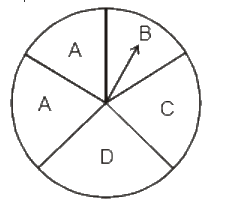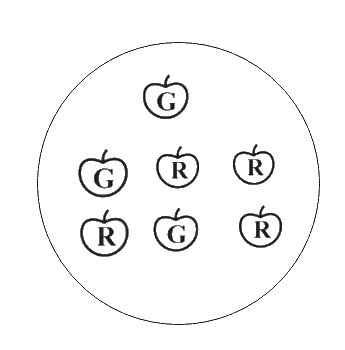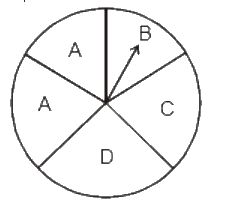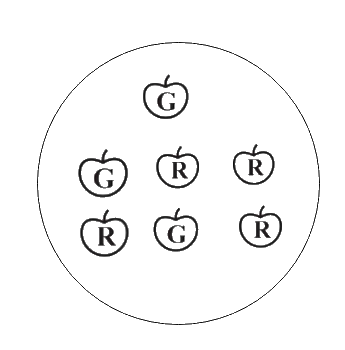# Excercise 5.3 Data Handling- NCERT Solution Class 8

Go back to  'Data Handling'

## Chapter 5 Ex.5.3 Question 1

List the outcomes you can see in these experiments.

(a) Spinning a wheel

(b) Tossing two coins together### Solution

What is known?

Two different condition.

What is unknown?

Outcomes of the given conditions.

Reasoning:

All the possibilities of an event are known as its outcomes.

Steps:

(a) There are four letters $$A, B, C$$ and $$D$$ in a spinning wheel. So, there are $$4$$ outcomes.

(b) When two coins are tossed together there are four possible outcomes

$$HH, HT, TH, TT.$$

(Here $$HT$$ means head on first coin and tail on second coin and so on.)

## Chapter 5 Ex.5.3 Question 2

When a die is thrown, list the outcomes of an event of getting:

(i)  (a) a prime number

(b) not a prime number

(ii) (a) a number greater than $$5$$

(b) a number not greater than $$5$$

### Solution

What is known?

A situation in which a die is thrown.

When a dice is thrown,the possible outcomes are  $$1,2,3,4,5$$ and $$6.$$

What is unknown?

Outcomes of the situation under given conditions.

Reasoning:

All the possibilities of an event are known as its outcomes.

Steps:

(i)

(a) Outcomes of event of getting a prime number are $$2, 3$$ and $$5.$$

(b) Outcomes of event of not getting a prime number are $$1, 4$$ and $$6.$$

(ii)

(a) Outcomes of event of getting a number greater than $$5$$ is $$6.$$

(b) Outcomes of event of not getting a number greater than $$5$$ are $$1, 2, 3, 4$$ and $$5.$$

## Chapter 5 Ex.5.3 Question 3

Find the:

(a) Probability of the pointer stopping on $$D$$ in (Question 1 (a))?

(b) Probability of getting an ace from a well shuffled deck of $$52$$ playing cards?

(c) Probability of getting a red apple? (See figure alongside)### Solution

What is known?

(i)  Spinning wheel image

(ii) Deck of $$52$$ playing cards

(iii) Image depicting Red and Green apples

What is unknown?

(i) Probability of the pointer stopping on $$D.$$

(ii) Probability of getting an ace from a well shuffled deck of $$52$$ playing cards.

(iii) Probability of getting a red apple

Reasoning:

Probability

\begin{align}= \! \frac{{{\text{Number of favourable outcomes}}}}{{{\text{Total number of outcomes}}}}\end{align}

Steps:

(a) In a spinning wheel, there are five pointers $$A, A, B, C, D.$$ So, there are five outcomes. Pointer stops at $$D$$ which is $$1$$ outcome. So, the probability of the pointer stopping on \begin{align}D = \frac{1}{5}\end{align}(b) There are $$4$$ aces in a deck of $$52$$ playing cards. So, there are $$4$$ events of getting an ace.

So, probability of getting an ace \begin{align} = \frac{4}{{52}} = \frac{1}{{13}}\end{align}

(c) Total number of apples $$= 7$$

Number of red apples $$= 4$$

So, probability of getting red apple \begin{align} = \frac{4}{7}\end{align}## Chapter 5 Ex.5.3 Question 4

Numbers $$1$$ to $$10$$ are written on ten separate slips (one number on one slip), kept in a box and mixed well. One slip is chosen from the box without looking into it.

What is the probability of:

(i)  getting a number $$6?$$

(ii)  getting a number less than $$6?$$

(iii) getting a number greater than $$6?$$

(iv)  getting a number less than $$6?$$

(v) getting a $$1-$$digit number?

### Solution

What is known?

Numbers $$1$$ to $$10$$ are written on ten separate slips (one number on one slip), kept in a box and mixed well

What is unknown?

(i) Probability of getting a number $$6$$

(ii) Probability of getting a number less than $$6$$

(iii) Probability of getting a number greater than $$6$$

(iv) Probability of getting a number less than $$6$$

(v) Probability of getting a $$1-$$digit number

Reasoning:

Probability\begin{align} = \frac{{{\text{Number of favourable outcomes}}}}{{{\text{Total number of outcomes}}}}\end{align}

Steps:

(i) Outcome of getting a number $$6$$ from ten separate slips is $$1$$. Therefore, probability of getting a number \begin{align}6 = \frac{1}{{10}}\end{align}

(ii) Numbers less than $$6$$ are $$1, 2, 3, 4$$ and $$5$$. So, there are $$5$$ outcomes. Therefore, probability of getting a number less than

\begin{align} 6 = \frac{5}{{10}} = \frac{1}{2} \end{align}

(iii) Number greater than 6 are $$7, 8, 9, 10.$$ So there are $$4$$ possible outcomes. Therefore, probability of getting a number greater than

\begin{align} 6 = \frac{4}{{10}} = \frac{2}{5} \end{align}

(iv) One-digit numbers are

$$1, 2, 3, 4, 5, 6, 7, 8, 9$$ out of ten.

Therefore, probability of getting a $$1$$-digit number

\begin{align} = \frac{9}{{10}} \end{align}

## Chapter 5 Ex.5.3 Question 5

If you have a spinning wheel with $$3$$ green sectors, $$1$$ blue sector and $$1$$ red sector, what is the probability of getting a green sector?

What is the probability of getting a non-blue sector?

### Solution

What is known?

Spinning wheel with $$3$$ green sectors, $$1$$ blue sector and $$1$$ red sector

What is unknown?

i)  probability of getting a green sector

ii) probability of getting a non-blue sector

Reasoning:

Probability

\begin{align}= \frac{{{\text{Number of favourable outcomes}}}}{{{\text{Total number of outcomes}}}}\end{align}

Steps:

Total number of sectors $$= 5$$

Total number of green sectors $$= 3$$

Therefore, probability of getting a green sector\begin{align} = \frac{3}{5} \end{align}

Total number of blue sectors $$= 1$$

Total number of non-blue sectors $$= 5 – 1 = 4$$

Therefore, probability of getting a non-blue sector \begin{align} = \frac{4}{5}\end{align}

## Chapter 5 Ex.5.3 Question 6

Find the probability of the events given in Question $$2.$$

### Solution

What is known?

A situation in which a die is thrown.

What is unknown?

Probability of the events

Reasoning:

Probability

\begin{align} =\frac{{{\text{Number of favourable outcomes}}}}{{{\text{Total number of outcomes}}}} \end{align}

Steps:

When a die is thrown, there are total six outcomes, i.e., $$1, 2, 3, 4, 5$$ and $$6.$$

(i) (a) $$2, 3, 5$$ are prime numbers. So, there are $$3$$ outcomes out of $$6.$$

Therefore,probability of getting a prime number

\begin{align} = \frac{3}{6} = \frac{1}{2} \end{align}

(b) $$1, 4, 6$$ are not the prime numbers. So, there are $$3$$ outcomes out of $$6.$$

Therefore, probability of getting a prime number

\begin{align} = \frac{3}{6} = \frac{1}{2} \end{align}

(ii) (a) Only $$6$$ is greater than $$5.$$ So, there is one outcome out of $$6.$$

Therefore, probability of getting a number greater than

\begin{align} 5 = \frac{1}{6} \end{align}

(b) Numbers not greater than $$5$$ are $$1, 2, 3, 4$$ and $$5.$$ So there are $$5$$ outcomes out of $$6.$$

Therefore, probability of not getting a number greater than

\begin{align} 5 = \frac{1}{6} \end{align}

Related Sections
Related Sections
Instant doubt clearing with Cuemath Advanced Math Program